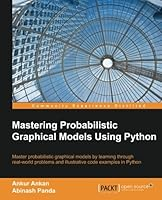# Mastering Probabilistic Graphical Models using Python## Book Description

Master probabilistic by learning through real-world problems and illustrative examples in Python

• Gain in-depth knowledge of Probabilistic Graphical Models
• Model time-series problems using Dynamic Bayesian
• A practical guide to help you apply PGMs to real-world problems

### Who This Book Is For

If you are a researcher or a machine learning enthusiast, or are working in the data science field and have a basic idea of Bayesian learning or probabilistic graphical models, this book will help you to understand the details of graphical models and use them in your data science problems.

### What You Will Learn

• Get to know the basics of probability and graph
• Work with Markov networks
• Implement Bayesian networks
• Exact inference techniques in graphical models such as the variable elimination algorithm
• Understand approximate inference techniques in graphical models such as message passing algorithms
• Sampling algorithms in graphical models
• Grasp details of Naive Bayes with real-world examples
• Deploy probabilistic graphical models using various libraries in Python
• Gain working details of Hidden Markov models with real-world examples

### In Detail

Probabilistic graphical models is a technique in machine learning that uses the concepts of graph theory to concisely represent and optimally predict values in our data problems.

Graphical models gives us techniques to find complex patterns in the data and are widely used in the field of speech recognition, information extraction, image segmentation, and modeling gene regulatory networks.

This book starts with the basics of probability theory and graph theory, then goes on to discuss various models and inference algorithms. All the different types of models are discussed along with code examples to create and modify them, and also run different inference algorithms on them. There is an entire chapter that goes on to cover Naive Bayes model and Hidden Markov models. These models have been thoroughly discussed using real-world examples.

Chapter 1: Bayesian Fundamentals
Chapter 2: Markov Network Fundamentals
Chapter 3: Inference – Asking Questions to Models
Chapter 4: Approximate Inference
Chapter 5: Model Learning – Parameter Estimation in Bayesian Networks
Chapter 6: Model Learning – Parameter Estimation in Markov Networks
Chapter 7: Specialized Models

## Book Details

• Title: Mastering Probabilistic Graphical Models using Python
• Author: ,
• Length: 287 pages
• Edition: 1
• Language: English
• Publisher:
• Publication Date: 2015-08-03
• ISBN-10: 1784394688
• ISBN-13: 9781784394684